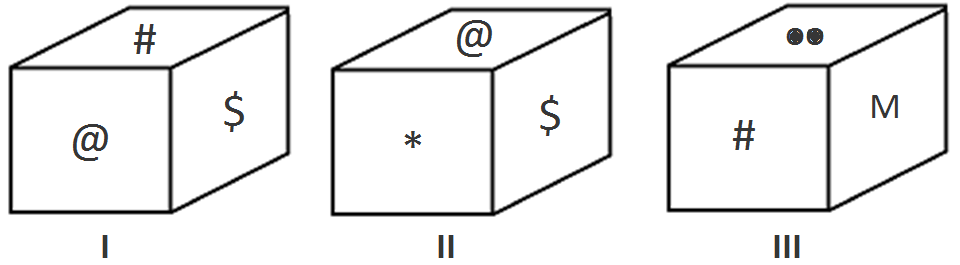Question of The Day15-10-2020

The following figure denotes the rotated position of a single solid cube. The various faces are marked with different symbols. Which of the following symbols is on the face opposite to the face having symbol ‘@’?Correct Answer : d ) M

Explanation :

To compare two different positions of dice to find out the numbers opposite to faces on the dice, we should follow the following steps:

• First, find the common number/symbol on both the positions of the dice.
• Then, starting from the common symbol, move in the clockwise direction in both the dices.
• While moving in the clockwise direction, note down the other numbers/symbols.
• Lastly, compare the numbers/symbols thus obtained. The next numbers after the common symbol in both the positions of the dice will be on the opposite faces of the dices.

NOTE: The above steps should be followed when there is only one common number/symbol on both the position of dice. If there are two common numbers/symbol on both the positions of dice, then the remaining symbols in both the positions will be opposite to each other.

Therefore, analyzing our dices, we get

For position I:

#, \$, @

The sequence of the numbers by the above logic will be

For position III:

#, Two dots, M

Thus, from the above logic we can say that symbol ‘M’ will be on the opposite face of ‘@’.

Hence, (d) is the correct answer.

Such type of question is asked in various government exams like SSC CGL, SSC MTS, SSC CPO, SSC CHSL, RRB JE, RRB NTPC, RRB GROUP D, UPSC CDS, UPSC NDA, etc.

Read Daily Current Affairs, Banking Awareness, Hindi Current Affairs, Word of the Day, and attempt free mock tests at PendulumEdu and boost your preparation for the actual exam.0